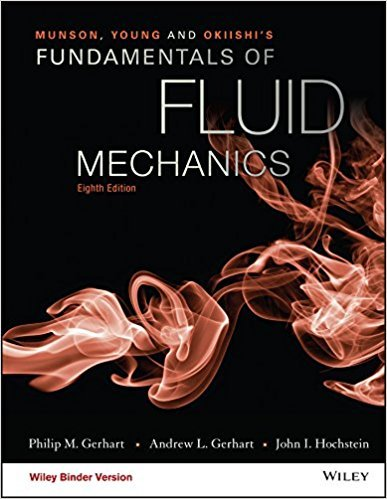×
Get Full Access to Fundamentals Of Fluid Mechanics - 8 Edition - Chapter 5.2.2 - Problem 5.43
Get Full Access to Fundamentals Of Fluid Mechanics - 8 Edition - Chapter 5.2.2 - Problem 5.43

×

# Air at T1 = 300 K, p1 = 303 kPa, and V1 = 0.5 m/s enters theVenturi shown in Fig. P5.43ISBN: 9781119080701 456

## Solution for problem 5.43 Chapter 5.2.2

Fundamentals of Fluid Mechanics | 8th Edition

• Textbook Solutions
• 2901 Step-by-step solutions solved by professors and subject experts
• Get 24/7 help from StudySoup virtual teaching assistantsFundamentals of Fluid Mechanics | 8th Edition

4 5 1 382 Reviews
31
5
Problem 5.43

Air at T1 = 300 K, p1 = 303 kPa, and V1 = 0.5 m/s enters theVenturi shown in Fig. P5.43. The air leaves at T2 = 220 K and p2 =101 kPa; A1 = 0.6 m2 and A2 = 1.0 m2. Calculate the horizontalforce required to hold the Venturi stationary.

Step-by-Step Solution:
Step 1 of 3

Biol 4305 February 23rd Clines  Mating with individuals nearby(isolation by distance) can cause alleles to flow across distances across time. [smallpox]  Can produc clines: o Continuous change in allele frequency along a geographical transect Study and estimates of gene flow  Directly o Radiotelemety o We have 3 rats o Measuring migration o Bad: cannot use it for all groups  Movement and migration does not = gene flow  Limited time frame  Long range/accidental movement ends up in gene flow  Indirectly o Based on the idea of population genetic structure o Assume they are in HW o Trying to see if allelelic few have a geographic component to their distribution o Expectations of genetypic freq  Distributions f heterozygosity (2pq)  Use FST (fixation index)  Measures the distribution of variation in allelic frequencies amoug & in between populations  Null---> no differences- all sub populations are connected OR gene flow is really high  We estimate FST= Ht-Hs (heterozygosity) all over/ Ht  Ht= total heterozygosity  Ha= individual sub population heterozygosity Empty P Q 2pq Subp1 .7 .3 .42 S

Step 2 of 3

Step 3 of 3

##### ISBN: 9781119080701

Since the solution to 5.43 from 5.2.2 chapter was answered, more than 1513 students have viewed the full step-by-step answer. This full solution covers the following key subjects: . This expansive textbook survival guide covers 112 chapters, and 1357 solutions. This textbook survival guide was created for the textbook: Fundamentals of Fluid Mechanics, edition: 8. Fundamentals of Fluid Mechanics was written by and is associated to the ISBN: 9781119080701. The full step-by-step solution to problem: 5.43 from chapter: 5.2.2 was answered by , our top Science solution expert on 03/16/18, 03:21PM. The answer to “Air at T1 = 300 K, p1 = 303 kPa, and V1 = 0.5 m/s enters theVenturi shown in Fig. P5.43. The air leaves at T2 = 220 K and p2 =101 kPa; A1 = 0.6 m2 and A2 = 1.0 m2. Calculate the horizontalforce required to hold the Venturi stationary.” is broken down into a number of easy to follow steps, and 51 words.

Unlock Textbook Solution